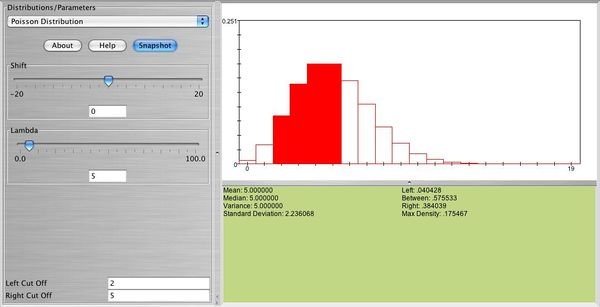# SOCR EduMaterials Activities Poisson Distribution

## This is an activity to explore the Poisson Probability Distribution.

• Exercise 1: Use SOCR to graph and print the distribution of a Poisson random variable with $$\lambda=2$$. What is the shape of this distribution?
• Exercise 2: Use SOCR to graph and print the distribution of a Poisson random variable with $$\lambda=15$$. What is the shape of this distribution? What happens when you keep increasing $$\lambda$$?
• Exercise 3: Let $$X \sim Poisson(5)$$. Find $$P(3 \le X < 10)$$, and $$P(X >10 | X \ge 4)$$.
• Exercise 4: Poisson approximation to binomial: Graph and print $$X \sim b(60, 0.02)$$. Approximate this probability distribution using Poisson. Choose three values of $$X$$ and compute the probability for each one using Poisson and then using binomial. How good is the approximation?

Below you can see the distribution of a Poisson random variable with $$\lambda=5$$. In this graph you can also see the probability that between 2 and 5 events will occur.• Exercise 5: People enter a gambling casino at a rate of 1 for every two minutes.
• What is the probability that no one enters between 12:00 and 12:05?
• What is the probability that at least 4 people enter the casino during that time?
• Exercise 6: Let $$X_1$$ denote the number of vehicles passing a particular point on the eastbound lane of a highway in 1 hour. Suppose that the Poisson distribution with mean $$\lambda_1=5$$ is a reasonable model for $$X_1$$. Now, let $$X_2$$ denote the number of vehicles passing a point on the westbound lane of the same highway in 1 hour. Suppose that $$X_2$$ has a Poisson distribution with mean $$\lambda_2= 3$$. Of interest is $$Y= X_1 + X_2$$, the total traffic count in both lanes in one hour. What is the $$P(Y < 5) ?$$